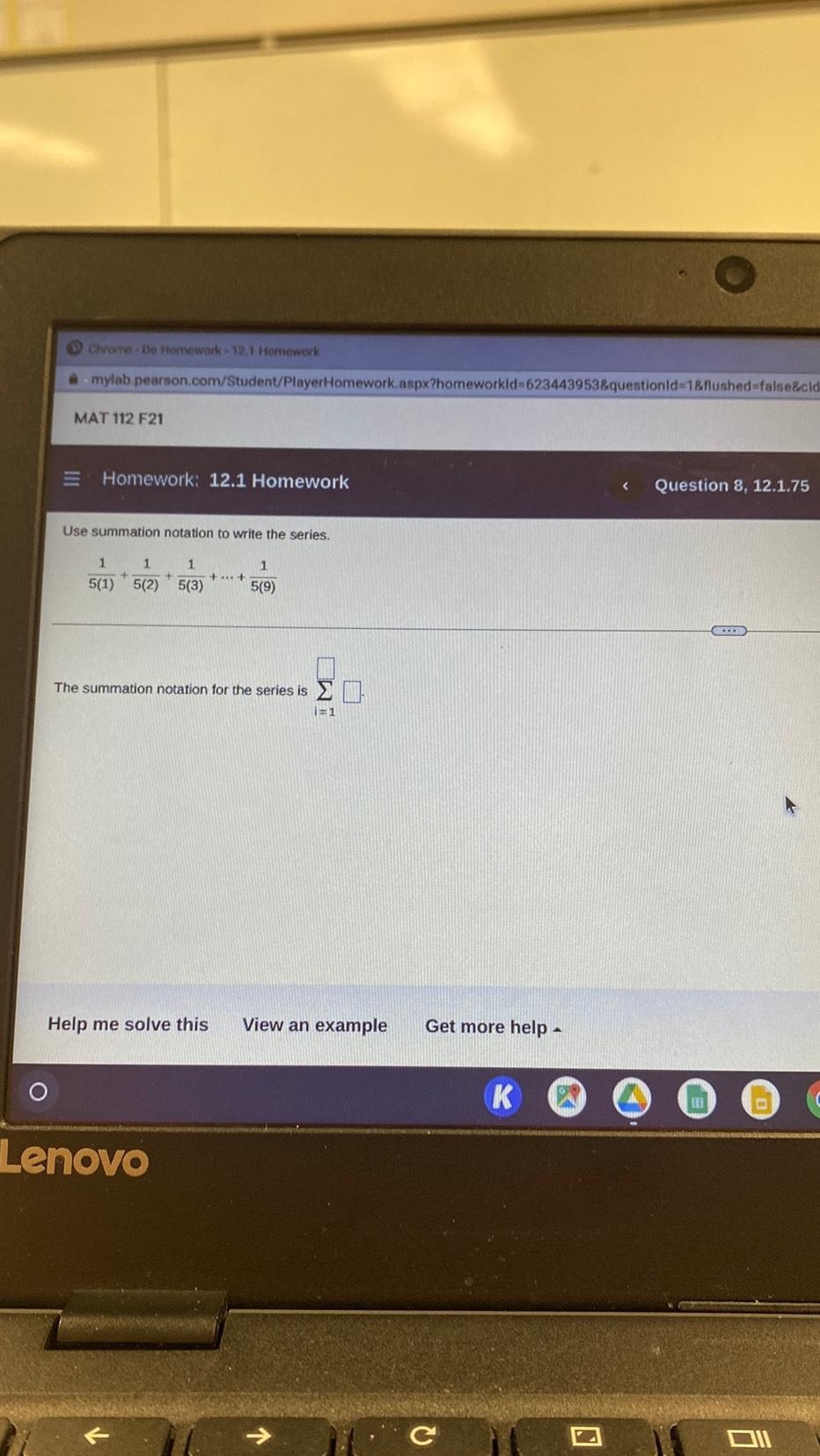Question:

# Use summation notation to write the series. 1/5(1) + 1/5(2) + 1/5(3) +-------=1/5(9) The summation notation for the series is ∑(i=1)Use summation notation to write the series. 1/5(1) + 1/5(2) + 1/5(3) +-------=1/5(9) The summation notation for the series is ∑(i=1)